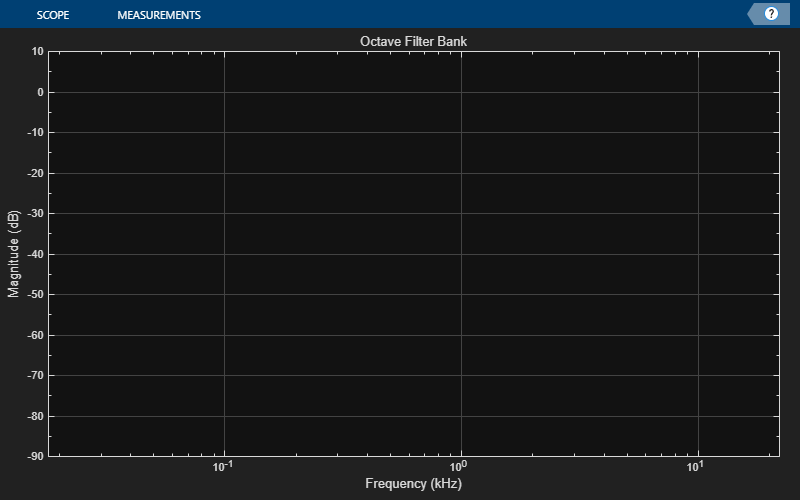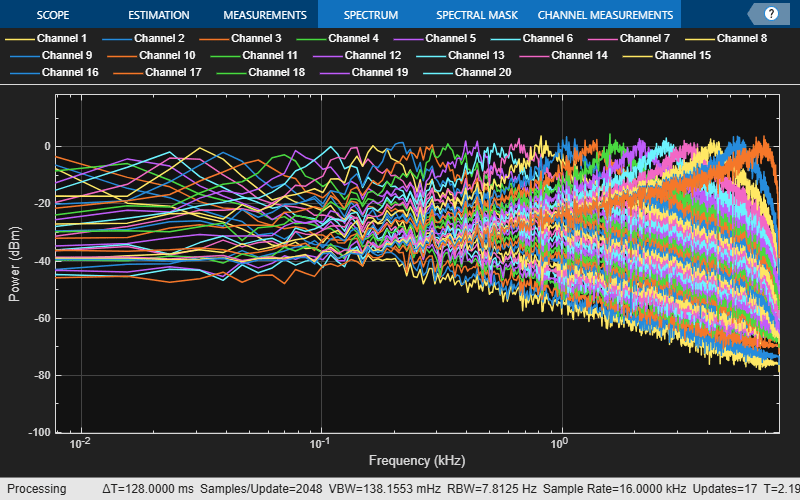# octaveFilterBank

Octave and fractional-octave filter bank

Since R2019a

## Description

`octaveFilterBank` decomposes a signal into octave or fractional-octave subbands. An octave band is a frequency band where the highest frequency is twice the lowest frequency. Octave-band and fractional octave-band filters are commonly used to mimic how humans perceive loudness.To apply a bank of octave-band or fractional octave-band filters:

1. Create the `octaveFilterBank` object and set its properties.

2. Call the object with arguments, as if it were a function.

## Creation

### Syntax

``octFiltBank = octaveFilterBank``
``octFiltBank = octaveFilterBank(bandwidth)``
``octFiltBank = octaveFilterBank(bandwidth,fs)``
``octFiltBank = octaveFilterBank(___,Name,Value)``

### Description

````octFiltBank = octaveFilterBank` returns an octave filter bank. The objects filters data independently across each input channel over time.```
````octFiltBank = octaveFilterBank(bandwidth)` sets the `Bandwidth` property to `bandwidth`.```
````octFiltBank = octaveFilterBank(bandwidth,fs)` sets the `SampleRate` property to `fs`.```
````octFiltBank = octaveFilterBank(___,Name,Value)` sets each property `Name` to the specified `Value`. Unspecified properties have default values.Example: ```octFiltBank = octaveFilterBank('1/2 octave','FrequencyRange',[62.5,12000])``` creates a ½ octave-band filter bank, `octFiltBank`, with bandpass filters placed between 62.5 Hz and 12,000 Hz.```

## Properties

expand all

Unless otherwise indicated, properties are nontunable, which means you cannot change their values after calling the object. Objects lock when you call them, and the `release` function unlocks them.

If a property is tunable, you can change its value at any time.

Filter bandwidth in octaves, specified as `'1 octave'`, `'2/3 octave'`, `'1/2 octave'`, ```'1/3 octave'```, `'1/6 octave'`, `'1/12 octave'`, `'1/24 octave'`, or `'1/48 octave'`.

Tunable: No

Data Types: `char` | `string`

Input sample rate in Hz, specified as a positive scalar.

Tunable: Yes

Data Types: `single` | `double`

Frequency range of the filter bank in Hz, specified as a two-element row vector of positive monotonically increasing values. The filter bank center frequencies are placed according to the `Bandwidth`, `RefererenceFrequency`, and `OctaveRatioBase` properties. Filters that have a center frequency outside `FrequencyRange` are ignored.

Tunable: No

Data Types: `single` | `double` | `int8` | `int16` | `int32` | `int64` | `uint8` | `uint16` | `uint32` | `uint64`

Reference frequency of the filter bank in Hz, specified as a positive integer scalar. The ANSI S1.11-2004 standard defines the center frequencies of the octave filters relative to the reference frequency. For more information, see Algorithms.

Tunable: No

Data Types: `single` | `double` | `int8` | `int16` | `int32` | `int64` | `uint8` | `uint16` | `uint32` | `uint64`

Order of the octave filters, specified as an even integer. The filter order applies to each individual filter in the filter bank.

Tunable: No

Data Types: `single` | `double` | `int8` | `int16` | `int32` | `int64` | `uint8` | `uint16` | `uint32` | `uint64`

Octave ratio base, specified as `10` or `2`. The octave ratio base determines the distribution of the center frequencies of the octave filters. The ANSI S1.11 standard recommends base 10. Base 2 is popular for music applications. Base 2 defines an octave as a factor of 2, and base 10 defines an octave as a factor of 100.3.

Tunable: No

Data Types: `single` | `double` | `int8` | `int16` | `int32` | `int64` | `uint8` | `uint16` | `uint32` | `uint64`

## Usage

### Syntax

``audioOut = octFiltBank(audioIn)``

### Description

example

````audioOut = octFiltBank(audioIn)` applies the octave filter bank on the input and returns the filtered output.```

### Input Arguments

expand all

Audio input to the octave filter bank, specified as a scalar, vector, or matrix. If specified as a matrix, the columns are treated as independent audio channels.

Data Types: `single` | `double`

### Output Arguments

expand all

Audio output from octave filter bank, returned as a scalar, vector, matrix, or 3-D array. The shape of `audioOut` depends on the shape of `audioIn` and the number of filters in the filter bank. If M is the number of filters, and `audioIn` is an L-by-N matrix, then `audioOut` is returned as an L-by-M-by-N array. If N is 1, then `audioOut` is a matrix.

Data Types: `single` | `double`

## Object Functions

To use an object function, specify the System object™ as the first input argument. For example, to release system resources of a System object named `obj`, use this syntax:

`release(obj)`

expand all

 `coeffs` Get filter coefficients `freqz` Compute frequency response `fvtool` Visualize filter bank `getBandedgeFrequencies` Get filter bandedges `getCenterFrequencies` Center frequencies of filters `getGroupDelays` Get group delays `info` Get filter information `isStandardCompliant` Verify octave filter bank is ANSI S1.11-2004 compliant
 `step` Run System object algorithm `release` Release resources and allow changes to System object property values and input characteristics `reset` Reset internal states of System object

## Examples

collapse all

Create a 1/3-octave filter bank for a signal sampled at 48 kHz. Set the frequency range to `[18 22000]` Hz.

```octFilBank = octaveFilterBank("1/3 octave",48000, ... FrequencyRange=[18 22000]); ```

Use `fvtool` to visualize the response of the filter bank. To get a high-resolution view on the lower frequencies, set the scale of the x-axis to `log` and `NFFT` to `2^16`.

```fvt = fvtool(octFilBank,"NFFT",2^16); set(fvt,FrequencyScale="log") zoom(fvt,[.01 24 -20 1]) ```Display the filter bank center frequencies.

```fc = getCenterFrequencies(octFilBank); cf = string(size(fc)); for ii = find(fc<1000) cf(ii) = sprintf("%.0f Hz",round(fc(ii),2,"significant")); end for ii = find(fc>=1000) cf(ii) = sprintf("%.1f kHz",fc(ii)/1000); end disp(cf) ```
``` Columns 1 through 7 "20 Hz" "25 Hz" "32 Hz" "40 Hz" "50 Hz" "63 Hz" "79 Hz" Columns 8 through 13 "100 Hz" "130 Hz" "160 Hz" "200 Hz" "250 Hz" "320 Hz" Columns 14 through 19 "400 Hz" "500 Hz" "630 Hz" "790 Hz" "1.0 kHz" "1.3 kHz" Columns 20 through 25 "1.6 kHz" "2.0 kHz" "2.5 kHz" "3.2 kHz" "4.0 kHz" "5.0 kHz" Columns 26 through 30 "6.3 kHz" "7.9 kHz" "10.0 kHz" "12.6 kHz" "15.8 kHz" Column 31 "20.0 kHz" ```

Process white Gaussian noise through the filter bank. Use a spectrum analyzer to view the spectrum of the filter outputs.

```sa = spectrumAnalyzer(SampleRate=16e3,... PlotAsTwoSidedSpectrum=false,... FrequencyScale="log"); for index = 1:500 x = randn(256,1); y = octFilBank(x); sa(y); end ```The `octaveFilterBank` enables good reconstruction of a signal after analyzing or modifying its subbands.

Read in an audio file and listen to its contents.

```[audioIn,fs] = audioread('RandomOscThree-24-96-stereo-13secs.aif'); sound(audioIn,fs)```

Create a default `octaveFilterBank`. The default frequency range of the filter bank is 22 to 22,050 Hz. Frequencies outside of this range are attenuated in the reconstructed signal.

`octFiltBank = octaveFilterBank('SampleRate',fs);`

Pass the audio signal through the octave filter bank. The number of outputs depends on the `FrequencyRange`, `ReferenceFrequency`, `OctaveRatioBase`, and `Bandwidth` properties of the octave filter bank. Each channel of the input is passed through a filter bank independently and is returned as a separate page in the output.

```audioOut = octFiltBank(audioIn); [N,numFilters,numChannels] = size(audioOut)```
```N = 1265935 ```
```numFilters = 10 ```
```numChannels = 2 ```

The octave filter bank introduces various group delays. To compensate for the group delay, remove the beginning delay from the individual filter outputs and zero-pad the ends of the signals so that they are all the same size. Use `getGroupDelays` to get the group delays. Listen to the group delay-compensated reconstruction.

```groupDelay = round(getGroupDelays(octFiltBank)); % round for simplicity audioPadded = [audioOut;zeros(max(groupDelay),numFilters,numChannels)]; for i = 1:numFilters audioOut(:,i,:) = audioPadded(groupDelay(i)+1:N+groupDelay(i),i,:); end```

To reconstruct the original signal, sum the outputs of the filter banks for each channel. Use `squeeze` to move the second channel from the third dimension to the second in the reconstructed signal.

```reconstructedSignal = squeeze(sum(audioOut,2)); sound(reconstructedSignal,fs)```

## Algorithms

The `octaveFilterBank` is implemented as a parallel structure of octave filters. Individual octave filters are designed as described by `octaveFilter`.

The ANSI S1.11-2004 standard  defines the center frequencies of the octave bands as

where:

• fr is the reference frequency, which is specified by the ReferenceFrequency property.

• G is the octave ratio, which is either 2 or 100.3, depending on value of the OctaveRatioBase property.

• b is the number of bands per octave, which is the inverse of the bandwidth of the filter in octaves. Specify the bandwidth with the Bandwidth property.

• k is any integer and represents the band number.

The center frequency definition differs depending on whether b is odd, such as for bandwidths of 1 octave or 1/3 octave, or even, such as for bandwidths of 1/2 octave or 1/6 octave. This ensures that the band edges of the whole octave band remain band edges for all of the fractional bands.Orfanidis, Sophocles J. Introduction to Signal Processing. Englewood Cliffs, NJ: Prentice Hall, 2010.

 Acoustical Society of America. American National Standard Specification for Octave-Band and Fractional-Octave-Band Analog and Digital Filters. ANSI S1.11-2004. Melville, NY: Acoustical Society of America, 2009.